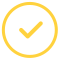effortlessly.

# Top-Rated Area Between Curves Calculator For Students

What is area between two curves? It refers to the integral of the absolute value of the difference between the two curves. These can be bound regions between intersecting points, enclosed areas, or areas between specified bounds.

In 2D geometry, the area between two curves shows the region occupied by the 2D figure.

## Area Between Two Curves Calculator: Definition

It is an online calculation tool that computes the area between curves (the enclosed shape). With this tool, you can save yourself the agonies of manually calculating extended functions, which may confuse you in the process.

Whether you want to find the area between two polar curves or desmos area between curves, this calculator will be a perfect pick for you. So, how exactly does the area between curves calculator work?

### How To Find Area Between Two Curves

When you want to calculate this, you will use the area between curves formula as illustrated below:

Consider,

P: y = f(x)

And Q: y = g(x)

Whereas X1 and X2 are the two limits,

Then the formula is,

Area Between Two Curves, A = ∫ba [f(x) − g(x)] dx

We can make use of the formula to calculate the area between the curves as in the examples below:

Example 1: Find the area between the two curves

f(x) = X2 + 3x + 1

g(x) = X2+2x+11

Solution

A = ∫ba [f(x) − g(x)] dx

A = ∫31 g(x) − f(x) dx

g(x) − f(x) = − x + 10

A = ∫31 (− x + 10) dx

= − x2/2 + 10x∣31

= − 9/2 + 30 − (−1/2 + 10)

= 16 units2

It is key to include units whenever you are calculating the area between curves. Therefore, you should develop a habit of writing "units2" when the units are not clear. You can practice this by the area between two curves examples found on our calculator.

### How To Use The Area Between Two Curves Calculator

This calculator has the average user in mind. Anyone can relate to the simplicity of this feature-rich calculator. Like the physical calculator, all you need to do is to enter the values and get your area between two curves within no time.

1. Enter functions; the larger & smaller values and the limit values
2. Proceed to click the ‘Calculate Area’ button just below the fields
3. You will then get your area in a new window

Using the find area between two curves calculator does not need any specialized knowledge. All you need to do is have the values at hand, key them in and get your results in a blink of an eye.

### Can You Do Math Homework?

Sometimes you may need more than a calculator to complete your math task. That is why we offer professional math homework help for all levels at affordable rates.

Graduate with an A+ in math with our expert help today!

Let’s make your assignment go away.Awesome. Now you'll never miss out.